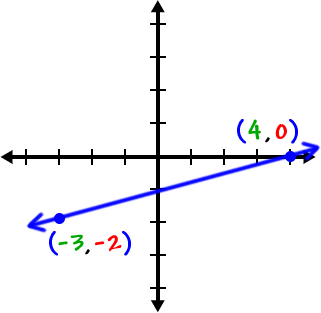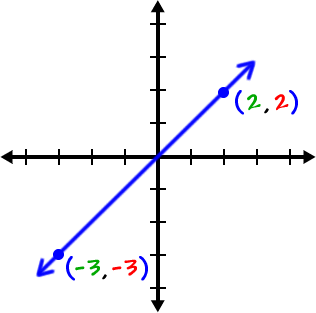One last thing on slopes of lines...

Remember that lines with positive slopes are ones that go uphill (as you go from left to right):

slope = 5slope = 1slope =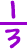Lines with negative slopes go downhill:

slope = -3slope = -1slope =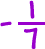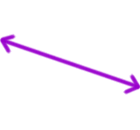Just for a little review, find the slopes of these lines: